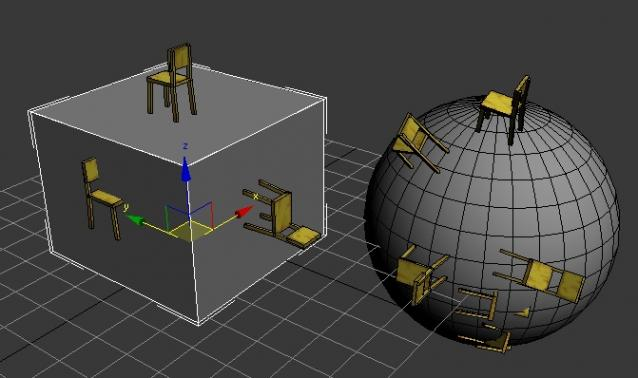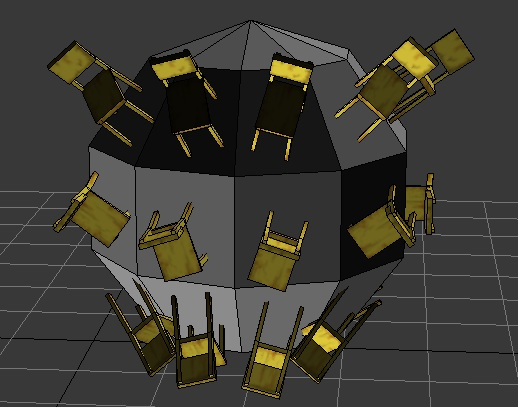# surface normal to quatI just can't figure this out. It works, but not perfect. (not on all faces)

``` theRay = mapScreenToWorldRay mouse.pos</br>
intersectArr = (intersectRayScene theRay)</br>
pos = intersectArr[intersectArr.count].pos</br>
dir = (intersectArr[intersectArr.count].dir)</br>
z=dir</br>
tempX=[1,0,0]</br>
y=normalize (cross z -tempX)</br>
x=normalize (cross y z)</br>
tm=matrix3 x y z theRay.pos</br>
rot = tm.rotation</br>
--also tested this line</br>
--rot = (quat dir.x dir.y dir.z 1</br>
obj.pos = pos obj.rotation = rot ```

any help appreciated!!

## Comment viewing options### So what's happening in these

So what's happening in these lines is you first create a world matrix, by giving the three axes and the position.

Now, the calculation for you y axis is a bit off.
First, you should change it to y = normalize(cross z tempX)

then, second of all, the calculation for the x axis is right but what happens on the left face is that the tempX axis and the z axis are eachothers exact opposite, leaving the calculated y vector to become 0;

this can be solved by simply checking for them to be either equal or eachothers exact opposite and using (0,1,0) as tempX instead in that case

i hope this makes sense
greetz FriesB### So what's happening in these

So what's happening in these lines is you first create a world matrix, by giving the three axes and the position.

Now, the calculation for you y axis is a bit off.
First, you should change it to y = normalize(cross z tempX)

then, second of all, the calculation for the x axis is right but what happens on the left face is that the tempX axis and the z axis are eachothers exact opposite, leaving the calculated y vector to become 0;

this can be solved by simply checking for them to be either equal or eachothers exact opposite and using (0,1,0) as tempX instead in that case

i hope this makes sense
greetz FriesB### problems with sphereHay, thanx for reply. it works perfect at a box, but have still problems with sphere's etc..

this is my code so far

```pos = &nbsp;intersectArr[intersectArr.count].pos
dir = &nbsp;(intersectArr[intersectArr.count].dir)
z=dir
tempX=[1,0,0]
y=normalize (cross z -tempX)
print y
if y == [1,0,0] do (
tempX = [0,0,1]
y=normalize (cross z -tempX)
if y == [0,1,0] do
(
y=normalize (cross z tempX)
)
)```

any thoughts?### try this:

try this:

pos =  intersectArr[intersectArr.count].pos
dir =  (intersectArr[intersectArr.count].dir)
z=dir
tempX=[1,0,0]

if dot z tempX == 1 do (
tempX = [0,1,0]
)
y=normalize (cross z tempX)
x=normalize (cross y z)